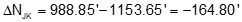### 3. Point-to-point computations

Point-to-point computations involve just two points

#### a. Forward Computation

A Forward Computation is used to calculate a point's coordinates from another set of coordinates using distance and direction data between them, Figure A-8.Equation A-1Equation A-2 Figure A-8 Forward computation

Equations A-1 and A-2 are a combination of the latitude and departure and coordinate equations from the Traverse Computations chapter:

• North Lat is (+), South Lat is (–)
• East Dep is (+), West Dep is (–)

DirAB can be either a bearing or azimuth:

• If a bearing (0° to 90°) is used you must manually determine the correct algebraic sign for the Lat and Dep based on quadrant.
• Using azimuths (0° to 360°) automatically results in correct signs.

#### b. Inverse Computation

An Inverse Computation determines the distance and direction between two coordinate pairs, Figure A-9.Figure A-9 Inverse computationEquation A-3Equation A-4Equation A-5Equation A-6

Coordinate differences, Δ’s, are the to point minus the from point: going from point C to point D means subtracting C's coordinates from D's.

The algebraic sign on β and the resulting direction depend on the quadrant of the line. A positive angle is clockwise (to the right); a negative angle is counterclockwise (to the left). Both are from the north or south end of the meridian, Figure A-10.Figure A-10 Direction quadrants
 Table A-1 Algebraic sign Direction Quadrant ΔN ΔE β Bearing Azimuth NE + + + N β E β SE - + - S |β| E 180°+β SW - - + S β W 180°+β NW + - - N |β| W 360°+β

When ΔN = 0, Equation A-7 has no solution. Technically division by 0 is undefined, but actually the result of any number divided by 0 is infinity. So what does this mean? In surveying terms when ΔN = 0 the entire line length is ΔE resulting in a due East or West line, Figure A-11.

 when ΔE is (+), Az = 90° when ΔE is (-), Az = 270°Figure A-11  When ΔN = 0

If you check the tangent of  90° and 270° on your calculator you'll get either an error or "undefined" response. Try tan(89.99999°); you'll get a huge number. Tan(90°) = tan(270°) = infinity.

#### c. Examples

##### (1)  Forward 1

Compute the coordinates of point R given the information in Figure A-12:Figure A-12 Forward Example 1

Because the bearing is South and East, the Lat is negative and Dep positive. From equations A-1 and A-2:##### (2)  Forward 2

Compute the coordinates of point R given the information in Figure A-13:Figure A-13 Forward Example 2

Because the direction is an azimuth, Equations A-1 and A-2 will automatically compute the correct signs for the Lat and Dep.##### (3) Inverse

What are the lengtha and azimuth from point J to K?

 Point North (ft) East (ft) J 1153.65 704.08 K 988.85 200.75

Draw a sketch to visualize the line, Figure A-14.Figure A-14 Inverse Example

Substitute the coordinates into Equations A-3 and A-4 (remember, it's to minus from):Use Equation A-5 to compute the length:Compute β, the angle from the meridian:Because ΔN is negative and ΔE is negative, the direction is in the South-West quadrant, so add 180° to β.